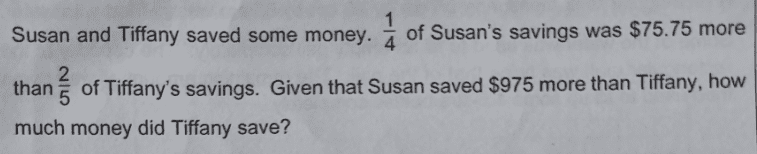# QuestionPls help. Thank you.

Source: assignment

I can only do by simultaneous equations.

Let S be the amount of money Susan saved.
Let T be the amount of money Tiffany saved.

(1) (1/4)S = 75.75 + (2/5)T

(2) S = T + 975

Substituting (2) into (1),
(1/4)(T + 975) = 75.75 + (2/5)T
(2/5 – 1/4)T = 975/4 – 75.75
((8 – 5)/20) T = 168
=> T = 168 (20/3) = \$1120 #

0 Replies 2 Likes ✔Accepted Answer